• 题意：求一元二次方程T*(C-N*T)取得最大值时，T的值是多少。 ac代码： //坑死，关键还是对题目的理解。 #include #include #include int main(){ int T; scanf("%d",&T); for(int i=1;i;i++){ long long...

E - E
Time Limit:2000MS     Memory Limit:32768KB     64bit IO Format:%lld & %llu
Submit Status

Description

A group of N Internet Service Provider companies (ISPs) use a private communication channel that has a maximum capacity of C traffic units per second. Each company transfers T traffic units per second through
the channel and gets a profit that is directly proportional to the factor T(C - T*N). The problem is to compute the smallest value of T that maximizes the total profit the N ISPs can get from using the channel.
Notice that N, C, T, and the optimalT are integer numbers.

Input

Input starts with an integer T (≤ 20), denoting the number of test cases.
Each case starts with a line containing two integers N and C (0 ≤ N, C ≤ 109).

Output

For each case, print the case number and the minimum possible value of T that maximizes the total profit. The result should be an integer.

Sample Input

6
1 0
0 1
4 3
2 8
3 27
25 1000000000

Sample Output

Case 1: 0
Case 2: 0
Case 3: 0
Case 4: 2
Case 5: 4
Case 6: 20000000
题意：求一元二次方程T*(C-N*T)取得最大值时，T的值是多少。
ac代码：
//坑死，关键还是对题目的理解。
#include<stdio.h>
#include<string.h>
#include<math.h>
int main(){
int T;
scanf("%d",&T);
for(int i=1;i<=T;i++){
long long N,C;
scanf("%lld%lld",&N,&C);
if(N==0){
printf("Case %d: %d\n",i,0);
continue ;
}
long long n,m;
float mid;
mid=(float)C/2/N;
n=floor(mid);//向下取整
m=ceil(mid);//向上取整
long long ans;
long long temp1=n*(C-N*n),temp2=m*(C-N*m);
if(temp1>=temp2){//尽量减少使用实数进行大小的判断。
ans=n;
}
else
ans=m;
printf("Case %d: %lld\n",i,ans);
}
return 0;
}



展开全文• 简单C语言小程序：根公式求一元二次方程式的根！ 一、算法的构思 一元二次方程的一般式：ax^2+bx+c = 0 我们知道，一元二次方程有解（根）的充要条件是：b^2-2ac>=0。如果不满足此关系式，那么方程无解。接着当...
简单C语言小程序：求根公式求一元二次方程式的根！
一、算法的构思
一元二次方程的一般式：ax^2+bx+c = 0
我们知道，一元二次方程有解（根）的充要条件是：b^2-4ac>=0。如果不满足此关系式，那么方程无解。接着当方程有解的时候又出现了两种情况：1.有两个重根（大小相等的根）或者两个大小不等的根，为了是程序更加完善还要考虑到a =0的情况，即此时不能看做一元二次方程而只能将其看作一元一次方程，本程序运用求根公式来实现功能，有兴趣的伙计可以试试下面给出的韦达定理实现功能。
求根公式：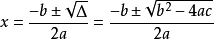扩展——韦达定理：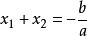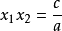二、全部代码
#include <stdio.h>
#include <math.h>
double a = 0, b = 0, c = 0, p, q;
int main(void)
{
printf("请分别输入a,b,c三个值并用空格隔开，按回车开始运算：\n");
scanf("%lf %lf %lf", &a, &b, &c);
if(a == 0 )
printf("此一元一次方程的解为x= %lf\n", -c/b);
else if (b*b-4*a*c<0)
printf("此方程无解\n");
else
{
p = -b/2*a;
q = (sqrt(b*b-4*a*c)/2*a);
printf("此一元二次方程的解为x1= %lf,x2= %lf\n该抛物线的对称轴为：= %lf\n最大/最小值为: = %lf", p+q,p-q, -b/2*a,(4*a*c-b*b)/4*a);
}
system("pause");
return 0;
}


三、给生成的程序添加一个漂亮的图标
我们知道，当C编译完成之后就会有一个默认的图标，很难看，如图：
那么我们如何可以给它换一个漂亮的图标呢？
1.首先，要去网上搜集几个你中意的ico图片文件来当他的图标。
2.在DEV C++左侧项目管理功能栏中找到项目，单击右键，弹出一个选择框，选择项目属性。
这时我们可以看到左下角有图标选项，点击浏览把从网上找来的ico文件选择进去再进行编译一次就可以了。
最后谢谢浏览，欢迎指正！


展开全文算法 c语言
• 输入一元二次方程的二次项、一次项及常数项，这样的话对应的一元二次方程表达式就确定了，点击【计算】，即可计算出该一元二次方程的根。 2. C#源程序 using System; using System.Collections.Generic; using...
目  录

1. 界面设计

2. C#源程序

1. 界面设计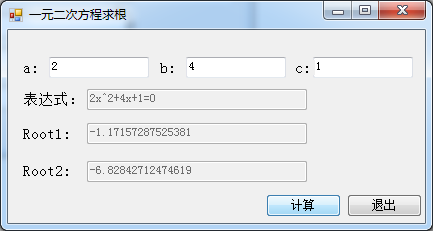界面说明：

输入一元二次方程的二次项、一次项及常数项，这样的话对应的一元二次方程表达式就确定了，点击【计算】，即可计算出该一元二次方程的根。

2. C#源程序

using System;
using System.Collections.Generic;
using System.ComponentModel;
using System.Data;
using System.Drawing;
using System.Linq;
using System.Text;
using System.Windows.Forms;

namespace 求一元二次方程的根
{
public partial class Form1 : Form
{
public Form1()
{
InitializeComponent();
}
private double[] Root2(double a, double b, double c)
{
double []Roots=new double;
double Delt=b * b - 4 * a * c;
if (Delt >= 0)
{
Roots = (-b + Math.Sqrt(Delt)) / 2 * a;
Roots = (-b - Math.Sqrt(Delt)) / 2 * a;
return Roots;
}
else
{
Roots = null;
return Roots;
}
}

private void button2_Click(object sender, EventArgs e)
{
Application.Exit();
}

private void button1_Click(object sender, EventArgs e)
{
if (textBox1.Text == string.Empty || textBox2.Text == string.Empty || textBox3.Text == string.Empty)
{
MessageBox.Show("请输入系数！"); return;
}
if (Root2(double.Parse(textBox1.Text), double.Parse(textBox2.Text), double.Parse(textBox3.Text)) == null)
{
MessageBox.Show("无实根啊！","提示",MessageBoxButtons.OK,MessageBoxIcon.Warning); return;
}
textBox4.Text = Root2(double.Parse(textBox1.Text), double.Parse(textBox2.Text), double.Parse(textBox3.Text)).ToString();
textBox5.Text = Root2(double.Parse(textBox1.Text), double.Parse(textBox2.Text), double.Parse(textBox3.Text)).ToString();
}
string a, b, c;
private void textBox1_TextChanged(object sender, EventArgs e)
{
a = textBox1.Text.Trim();
textBox6.Text = a + "x^2+" + b + "x+" +c+"=0";
}

private void textBox3_TextChanged(object sender, EventArgs e)
{
c = textBox3.Text.Trim();
textBox6.Text = a + "x^2+" + b + "x+" + c + "=0";
}

private void textBox2_TextChanged(object sender, EventArgs e)
{
b = textBox2.Text.Trim();
textBox6.Text = a + "x^2+" + b + "x+" + c + "=0";
}
}
}


展开全文• 一元二次方程 形态：ax²+bx+c=0 (a≠0) 根公式： x=(-b±(b²-4ac)^(1/2))/(2a) 当b²-4ac>0时有两个不相等的实根 当b²-4ac=0时有两个相同的实根 当b²-4ac（虚根） 注：在正式学习高数之前，对于复数只...
一元二次方程

形态：ax²+bx+c=0   (a≠0)

求根公式：
x=(-b±(b²-4ac)^(1/2))/(2a)
当b²-4ac>0时有两个不相等的实根
当b²-4ac=0时有两个相同的实根
当b²-4ac<0时没有实根，但有两个共轭的复根（虚根）

注：在正式学习高数之前，对于复数只需要知道i²=-1即可

韦达定理（x1、x2为方程的两个根）：x1+x2=-(b/a),x1*x2=(c/a)

注：在解一元二次方程求根时，当条件允许时，可尽量使用十字相乘法求根。但如果方程无实根，则应用求根公式求虚根。

例题1：
x²+x-2=0
解：
使用十字相乘法得：
(x-1)(x+2)=0
则方程的两个根为：x1=1,x2=-2。
或
使用求根公式得：
x=(-1±(1²-4*1*(-2))^(1/2))/(2*1)
=(-1±3)/2
则方程的两个根为：x1=1,x2=-2。

例题2：
x²+6x+13=0
解：
b²-4ac=36-52=-16<0
因此方程无实根。则使用求根公式求其虚根：
x=(-6±(-16)^(1/2))/2
则方程的两个虚根为：x1=-3+2i,x2=-3-2i。

注：-16可写为16i²(i²即-1)则开平方根得4i

一元二次函数

形态1（通式）：y=ax²+bx+c   (a≠0)
形态2（顶点式）：y=a(x+b/(2a))²+(4ac-b²)/(4ac)   (a≠0)

注：对于一元二次函数，只要获得其顶点式，就可直接获取其图像几乎所有的性质。因此当遇到通式形态的一元二次函数，应优先将之转换为顶点式。

图像性质：
开口：由a决定，当a为正数时开口向上，a为负数时开口向下。
顶点：(-b/(2a),(4ac-b²)/(4ac))
对称轴：x=-b/(2a)
最大值：当开口向上时无最大值，当开口向下时最大值为(4ac-b²)/(4ac)
最小值：当开口向下时无最小值，当开口向上时最小值为(4ac-b²)/(4ac)
单调性：当开口向上时，(-∞,-b/(2a))单调递减，(-b/(2a),+∞)单调递增；当开口向下时，(-∞,-b/(2a))单调递增，(-b/(2a),+∞)单调递减。
交点个数（与x轴）：
当b²-4ac>0时有两个交点
当b²-4ac=0时有一个交点
当b²-4ac<0时无交点
注：交点的横坐标计算方式及解一元二次方程，纵坐标恒为零

例题：
y=x²+x+1 求该函数的一系列性质
解：
使用公式求得其顶点式为：
y=(x+1/2)²+3/4
则其开口向上，最小值为3/4
对称轴为x=-1/2
在(-∞,-1/2)单调递减，在(-1/2,+∞)单调递增
b²-4ac=-3<0。和x轴无交点

指数

形态：a^n

公式：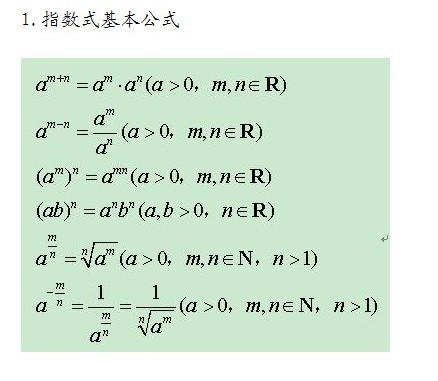例题：
(x^5)(x^6)
=x^11

对数

公式：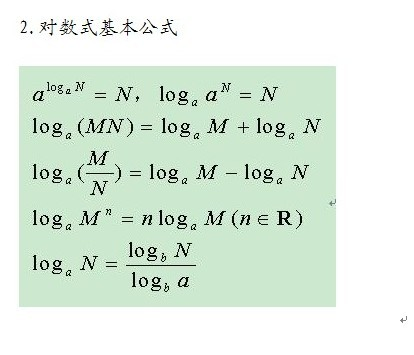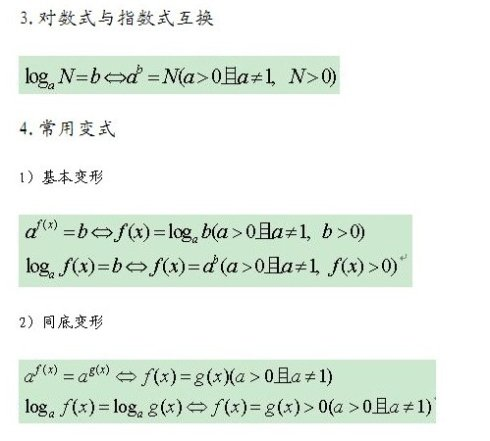例题：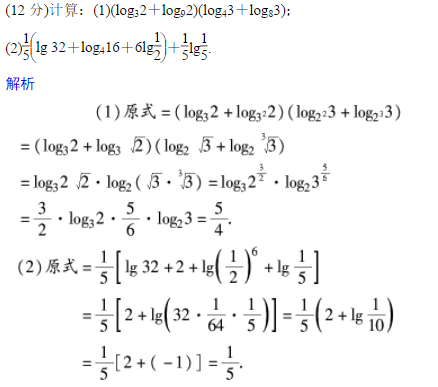展开全文数学
• a = input('Enter A: '); b = input('Enter B: '); c = input('Enter C: '); d= b^2 - 4 * a * c; if d> 0  x1 = (-b + sqrt(d)) / (2*a);  x2 = (-b - sqrt(d)) / (2*a);...fprintf('x1matlab input c
• 1.用函数实现求一元二次方程ax^2+bx+c=0的根，要求二次方程的系数a，b，c由键盘输入。 #include "iostream" #include "math.h" using namespace std; void getValue(double a,double b,double c){ if(b*b-4*a*c<...c++
• 一、用函数实现求一元二次方程ax^2+bx+c=0的根，要求二次方程的系数a、b、c分别从键盘输入: #include <iostream> #include"math.h" using namespace std; int panduan(double, double, double); void main...c++
• 其实现在网络上关于遗传算法求一元函数最大值的代码已经很多了，本文也是借鉴了他们的经验、算法思想、代码等撰写出来的，只是在一讨论中听了别人对于其中算法的看法，认为其中基于轮盘赌法的非线性选择有局限性，...算法 matlab 函数
• 将函数值y视为关于x的一元二次方程的系数，然后用判别式大于等于0求解。  用换元法化成二次函数的问题
• ﻿﻿ ... 烟台大学计算机与控制学院 All ringts reserved.... 文件名称：OJ总结－三角形面积-gyy ... 求一元二次方程ax2+bx+c=0的解。a,b,c为任意实数。 输入数据有一行，包括a b c的。按以下格式输出方 程的根x1和xc语言 函数
• #include "stdio.h" #define F(x) (x*x+2*x+3) //目标函数 #define dF(x) (2*x+2) //函数一阶导 #define dF_(x) 2 //函数二阶导 #define update(x) (x-dF(x)/dF_(x)) //x更新规则 void main() ...
• 经过转换之后就是杯中热水的高度的一元二次方程，极值。 注意点： 函数的频繁调用会造成精度损失 #define pi acos(-1.0) #define g(h) h/H*(R-r)+r #define V(h) pi/3 * h * ( ss*ss+ss*r+r*r) - v...
• 一元函数 对于如下图所示的一元函数求解其在区间[0,7]内的最大值有多种方式。在本文中分享的是用一种启发式算法——遗传算法来完成这项工作。 大家对遗传算法不了解的话可以戳这里看简介。 首先介绍我们的主角，也...算法
• maximum：合集中的最大值； range：从最小值到最大值的范围。 离中趋势度量（也称为离散程度度量），例如range，可以帮助我们确定值的分布情况。 01 确定三个值中的最小值 我们来编写程序确定三个值中的最小值。...
• 问题：遗传算法求解区间[0, 31]上的二次函数f(x) = x ^ 2的最大值 #include <bits/stdc++.h> using namespace std; const int maxn = 10; //种群数量 const int bit = 5; const int iteration = 200; //...
• 一、问题描述用GA求解一元函数的最大值：f(x) = x sin(10πx) + 2.0, x∈[-1,2] 、编码变量x可以视为遗传算法的表现型形式，我们采用进制编码形式。如果设定求解精度要精确到6位小数，由于区间长度为3，故将区间人工智能 遗传算法 优化问题 c语言
• 问题 A: 例题4-1 一元二次方程根 时间限制: 1 Sec 内存限制: 12 MB题目描述 求一元二次方程ax2+bx+c=0的根，三个系数a, b, c由键盘输入，且a不能为0，但不保证b2-4ac&gt;0。 程序中所涉及的变量均为...
• 思路：对a进行分情况讨论，a == 0，那么就是一元二次方程最值; a != 0，求导极值点。 代码如下： #include #include #include using namespace std; double a,b,c,d,l,r; double fun(double x) { return...
• 问题：二元函数的最大值 f(x)=x^2,x[0,31] 遗传算法实现步骤： 1.编码 编码方法包括：进制编码法、浮点编码法、符号编码法 这里使用进制编码。由于变量的最大值31，固采用5个位即可表达全部的值 x=...
• 牛顿迭代法（Newton’s method）又称为牛顿-拉夫逊方法（Newton-Raphson method），它是牛顿在17 世纪提出的一种在实数域和复数域上近似求解方程的方法。...牛顿迭代法是方程根的重要方法之一，其最大优点是在方
• MATLAB求一元函数极值命令介绍此次用的是机房的R2010版本命令： 1、x=fminbnd(fun,x1,x2) 出...3、fminbnd算法基于黄金分割法和二次插值法，要求fun必须是连续函数 4、fun可以用字符串定义也可以用inline函数定义matlab
• #include using namespace std; int main() { int a,b,c; cin>>a>>b>>c; if(a>b&&b>c) cout  else if(b>a&&b>c) cout else cout return 0; }
• 这里使用一元二次方程为例。// 梯度下降算法一元凸函数的最优解(极值点) // 梯度下降算法 X(n+1) = X(n) - ηf'(X) // 设一元凸函数 y = ax^2 + bx + c (a &gt; 0) // 1.构造一元凸函数 fl...梯度下降
• 已知四元一方程x<sub>1</sub>+2x<sub>2</sub>+3x<sub>3...f=2<sup>x<sub>1</sub></sup>+3<sup>x<sub>2</sub></sup>+4<sup>x<sub>3</sub></sup>+5<sup>x<sub>4</sub></sup>取最大值的解。解题的python程序代码。python
• C语言作业1-顺序选择结构设计-3三个数比较出最大值 问题描述： 输入并运行下面程序： 三个整数a，b，c，由键盘输入这三个数,三个数中最大的值。 代码实现： #include&lt;stdio.h&gt; #include&lt;...
• a=int(input('输入一个数')) b=int(input('输入一个数')) ...a: # 当b大于最大值时 更换最大值 max=b if c&gt;b: # 当c大于最大值时 更换最大值 max=c print('max=',max) 分析: 假设a为最大值,当b比...
• 求解f(x) = x + 10sin(5x) + 7cos(4x)在给定区间上的最大值，通过简单修改，即可求解任意函数在任意给定区间上的极大值/极小值。遗传算法是一种通用的优化算法。代码放在github上了。算法 python
• 问题：y=xsin⁡10x+xcos⁡2xy=x\sin{10x}+x\cos{2x}y=xsin10x+xcos2x在[0,5][0, 5][0,5]上的最大值。 解题步骤： 1、随机生成种群poppoppop，每个种群中含有100100100条染色体。每个染色体上含有10个基因（以...遗传算法 最值问题 python
• 啊第一次在csdn上写东西，在前些时候优化方法的计算实习老师留了一些上机作业，第一道题目是用不同的优化算法结合最速下降法求解二次函数。 二次函数及梯度的生成 先贴代码/matlab: function Function_value = ...matlab 优化算法 共轭梯度法 最速下降法...